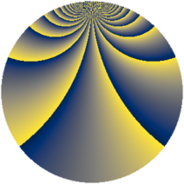# Properties

 Label 1104.2.bcLevel $1104$ Weight $2$ Character orbit 1104.bc Rep. character $\chi_{1104}(17,\cdot)$ Character field $\Q(\zeta_{22})$ Dimension $460$ Sturm bound $384$

# Related objects

## Defining parameters

 Level: $$N$$ $$=$$ $$1104 = 2^{4} \cdot 3 \cdot 23$$ Weight: $$k$$ $$=$$ $$2$$ Character orbit: $$[\chi]$$ $$=$$ 1104.bc (of order $$22$$ and degree $$10$$) Character conductor: $$\operatorname{cond}(\chi)$$ $$=$$ $$69$$ Character field: $$\Q(\zeta_{22})$$ Sturm bound: $$384$$

## Dimensions

The following table gives the dimensions of various subspaces of $$M_{2}(1104, [\chi])$$.

Total New Old
Modular forms 2040 500 1540
Cusp forms 1800 460 1340
Eisenstein series 240 40 200

## Trace form

 $$460q + 9q^{3} + 22q^{7} - 9q^{9} + O(q^{10})$$ $$460q + 9q^{3} + 22q^{7} - 9q^{9} - 18q^{13} + 11q^{15} + 22q^{19} - 11q^{21} - 56q^{25} - 9q^{27} + 26q^{31} - 11q^{33} - 22q^{37} + 9q^{39} + 22q^{43} + 16q^{49} + 11q^{51} + 22q^{55} - 11q^{57} - 22q^{61} + 11q^{63} + 22q^{67} - 9q^{69} - 18q^{73} + 47q^{75} + 22q^{79} - 73q^{81} + 2q^{85} + 171q^{87} + 90q^{93} - 22q^{97} + 209q^{99} + O(q^{100})$$

## Decomposition of $$S_{2}^{\mathrm{new}}(1104, [\chi])$$ into newform subspaces

The newforms in this space have not yet been added to the LMFDB.

## Decomposition of $$S_{2}^{\mathrm{old}}(1104, [\chi])$$ into lower level spaces

$$S_{2}^{\mathrm{old}}(1104, [\chi]) \cong$$ $$S_{2}^{\mathrm{new}}(69, [\chi])$$$$^{\oplus 5}$$$$\oplus$$$$S_{2}^{\mathrm{new}}(138, [\chi])$$$$^{\oplus 4}$$$$\oplus$$$$S_{2}^{\mathrm{new}}(276, [\chi])$$$$^{\oplus 3}$$$$\oplus$$$$S_{2}^{\mathrm{new}}(552, [\chi])$$$$^{\oplus 2}$$